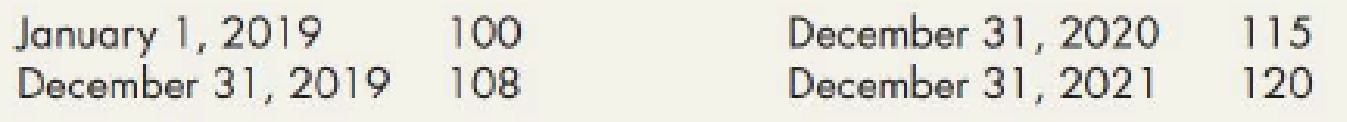Chapter 8, Problem 10P### Intermediate Accounting: Reporting...

3rd Edition
James M. Wahlen + 2 others
ISBN: 9781337788281

#### Solutions

Chapter
Section### Intermediate Accounting: Reporting...

3rd Edition
James M. Wahlen + 2 others
ISBN: 9781337788281
Textbook Problem
480 views

# Dollar-Value LIFO Retail The following information is obtained from Burger Company’s records. Burger uses the dollar-value LIFO retail method.The company adopted LIFO on January 1, 2019, when the cost and retail values of the inventory were $50,000 and$100,000, respectively. Burger experienced the following price indexes:Required:Compute the cost of the ending inventory for 2019, 2020, and 2021. Round the cost-to-retail ratio to 3 decimal places.

To determine

Calculate the cost of ending inventory for 2019, 2020, and 2021 using the dollar-value LIFO retail inventory method.

Explanation

Dollar-Value-LIFO: This method shows all the inventory figures at dollar price rather than units. Under this inventory method, the units that are purchased last are sold first. Thus, it starts from the selling of the units recently purchased and ending with the beginning inventory.

Calculate the cost of ending inventory for 2019, 2020, and 2021 using the dollar-value LIFO retail inventory method.

For the year 2019:

Step 1: Calculate the amount of estimated ending inventory at retail.

 B Company Ending Inventory Under DVL Retail Method For the Year 2019 Details Cost ($) Retail ($) Beginning inventory 50,000 100,000 Add:  Net purchase 200,000 420,000 Net additional markups 20,000 Less:  Net markdowns 0 (10,000) Goods available for sale – Excluding beginning inventory 200,000 430,000 Goods available for sale – Including beginning inventory 250,000 530,000 Less:  Net sales (400,000) Estimated ending inventory at retail for 2019 $130,000 Table (1) Step 2: Calculate ending inventory at retail at base-year prices. Ending inventory at retail at base-year prices }=[ Ending inventory at retail×(Price index on 1/1/19Price index on 31/12/19)]=[$130,000×(100108)]=$120,370 Step 3: Calculate inventory change at retail at base year prices. Inventory change at retail at base-year prices}(Ending inventory at retail at base-year pricesBeginning inventory at retail)=($120,370$100,000)=$20,370

Step 4: Calculate the change at relevant current costs.

Change at relevant current costs =[Inventory change at retail at base-year prices×(Price index on 31/12/19Price index on 1/1/19)×Cost-to-retail ratio]=[$20,370×(108100)×.465]=$10,230

Step 5: Calculate ending inventory at cost.

Ending inventory at cost = (Base-year layer + 2019 layer)=[$50,000(Beginning inventory for 2019)+$10,230(Change at current cost)]=$60,230 Hence, the ending inventory at cost for 2019 is$60,230.

Working note 1:

Calculate cost-to-retail ratio.

Cost-to-retail ratio= (Beginning inventory for costBeginning inventory for retail)=($50,000$100,000)=.5

Working note 2:

Calculate cost-to-retail ratio.

Cost-to-retail ratio= (Goods available for sale at retail excluding beginning inventoryGoods available for sale at retail excluding beginning inventory)=($200,000$430,000)=.465

For the year 2020:

Step 1: Calculate the amount of estimated ending inventory at retail.

 B Company Ending Inventory Under DVL Retail Method For the Year 2020 Details Cost ($) Retail ($) Beginning inventory 60,230 130,000 Add:  Net purchase 250,000 550,000 Net additional markups 30,000 Less:  Net markdowns 0 (40,000) Goods available for sale – Excluding beginning inventory 250,000 540,000 Goods available for sale – Including beginning inventory 310,230 670,000 Less:  Net sales (600,000) Estimated ending inventory at retail for 2020 $70,000 Table (2) Step 2: Calculate ending inventory at retail at base-year prices. Ending inventory at retail at base-year prices }=[ Ending inventory at retail×(Price index on 1/1/19Price index on 31/12/20)]=[$70,000×(100115)]=$60,870 Step 3: Calculate inventory change at retail at base year prices. Inventory change at retail at base-year prices}(Ending inventory at retail at base-year prices for 2020Ending inventory at retail at base-year prices for 2019)=($60,870$120,370)=$59,500

Step 4: Calculate the total change at relevant current costs.

(i)

Calculate the change at relevant current costs for 2019 (2019 layer).

Change at relevant current costs =[Inventory change at retail at base-year prices×(Price index on 31/12/19Price index on 1/1/19)×Cost-to-retail ratio]=[\$20,370×(108100)×

### Still sussing out bartleby?

Check out a sample textbook solution.

See a sample solution

#### The Solution to Your Study Problems

Bartleby provides explanations to thousands of textbook problems written by our experts, many with advanced degrees!

Get Started

#### Find more solutions based on key concepts